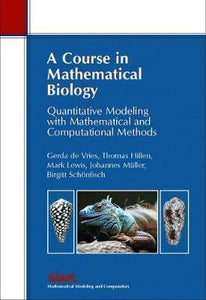# A Course in Mathematical Biology by Vries, Gerda de

• R 250.00
Unit price per
Tax included.

ISBN: 9780898716122

Society for Industrial & Applied Mathematics,U.S. | 01 January 2006

Paperback | 321 pages

This is the only book that teaches all aspects of modern mathematical modeling and that is specifically designed to introduce undergraduate students to problem solving in the context of biology. Included is an integrated package of theoretical modeling and analysis tools, computational modeling techniques, and parameter estimation and model validation methods, with a focus on integrating analytical and computational tools in the modeling of biological processes. Divided into three parts, it covers basic analytical modeling techniques; introduces computational tools used in the modeling of biological problems; and includes various problems from epidemiology, ecology, and physiology. All chapters include realistic biological examples, including many exercises related to biological questions. In addition, 25 open-ended research projects are provided, suitable for students. An accompanying Web site contains solutions and a tutorial for the implementation of the computational modeling techniques. Calculations can be done in modern computing languages such as Maple, Mathematica, and MATLAB?.